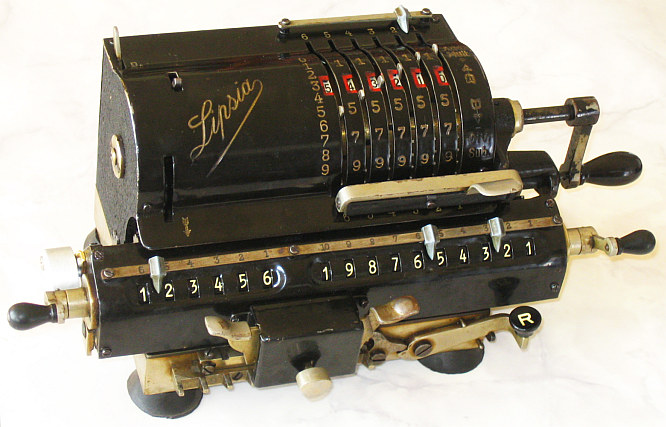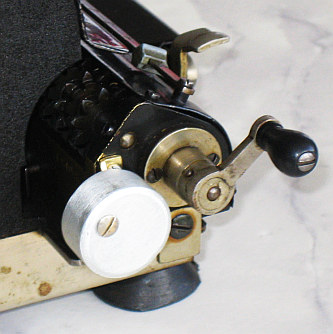previous <<==>> next

LIPSIA
MODEL  11RHave a look at the Restoration Procedure ...

 ``` TECHNICAL DATA of the Mechanical Calculator L I P S I A » Model 11R « ***************************************************************************** Serial Number: 36059 Dimensions: (ca.) Width = 11 " / 28 cm Depth = 5_1/2 " / 14 cm Height = 4_3/4 " / 12 cm Weight: (ca.) 9 lbs / 4.1 kg Mechanics: Pin-Wheel / Sprossenrad 10s Carry Mechanism Counter Stepping Mechanism + Animation Functions: Add, Subtract, Multiply, Divide Back-Transfer / Rueckuebertragung Registers: Input = 6 Decimals Counter = 6 Decimals Arithmetic = 10 Decimals Manufacturer: LIPSIA RECHENMASCHINENFABRIK, Otto Holzapfel & Cie, Leipzig / Germany 1950 H O W T O U S E the LIPSIA Model 11R ******************************************** BASIC SETTINGS: =============== (a) Crank: ---------- For addition (+) the crank is turned clockwise; for subtraction (-) the crank is turned counter-clockwise. In idle position the crank is down & locked. To make one or more turns with the crank, pull out the handle to unlock. When finished the turn(s), let the handle snap into the lock again. The locked down position only makes other functions accessible. REMARK: Every started turn has to be finished completely! Accidentally started turns are correctible somehow. (b) Clearing Registers: ----------------------- The input can be cleared with the upper front lever by lifting it up (It is intended to use the thumb of the right hand for). The counter unit will be cleared with the crank on the left side of the carriage turning clockwise with the left hand. The arithmetic register will be cleared with the crank on the right side of the carriage turning counter-clockwise with the right hand. (c) Shifting the Carriage: -------------------------- Pushing the LEFT SILVER LEVER on front will move the carriage one step left - Pushing the RIGHT SILVER LEVER on front will move the carriage one step right. When the SIVER KNOB ON FRONT is lifted & hold, the carriage can be moved continuously right or left by hand. The left position "1" is the "Start Position" of the carriage. (d) Set Counting Direction with the Lever on Top: ------------------------------------------------- Set the TOP-LEFT-LEVER BACK: The counter and arithmetic unit are working in the same direction; In the opposite case [D = Division] both are working in opposit direction. (e) Back-Transfer: ------------------ For continuous multiplication the content of the arithmetic unit can be back transfered into the input register with this procedure: CLEAR the Input, PUSH & HOLD Lever (R) right on front w. left hand; CLEAR the Arithmetic-Unit [= Back-Transfer !]; Release Lever (R) ADDITION & SUBTRACTION: ======================= Example: 123 + 45 - 6 = 162 Clear input, counter and arithmetic units. ADD: Enter the first number (123) in the far right of the input unit. Make a positive (clockwise) turn with the crank to transfer the number into arithmetic unit. The counting unit displays the figure 1. Enter the second number (45). Make a positive (clockwise) turn with the crank to add the number. The arithmetic unit displays the intermediate sum (168) and the counting unit displays the figure 2. SUBTRACT: Enter the third number (6). Make a negative (counter-clockwise) turn with the crank. The arithmetic unit displays the result (162) and the counting unit is decreased by 1. REMARK: NEGATIVE RESULTS are displayed in the arithmetic unit as the COMPLEMENT of the next higher 10, 100, 1000, ... Example: -12 = 99...9988 MULTIPLICATION: =============== Example: 123 x 45 = 5535 Clear input, counter and arithmetic units. Enter the multiplicand (123) in the far right of the input unit. The multiplicator (45) has two digits, so the carriage is shifted to position 2. Make positive (clockwise) turns with the crank, until the first figure of the multiplicator (4) will appear in the 2nd position of the counter unit. Shift the carriage to position 1. Repeat making positive turns with the crank, until the second figure of the multiplicator (5) appears in the 1st position of the counter unit. The multiplication is done: The multiplicand (123) stays in the input unit, the multiplicator (45) in the counter and the result (5535) is in the arithmetic unit. DIVISION: ========= Example: 22 : 7 = 3.14285 Remainder 5 Division requires 3 steps: (1st) To Set the Dividend into Arithmetic Unit: ----------------------------------------------- For the maximum number of decimals, pull out the carriage to the far right position. Enter the dividend (22) in the far right of the input unit. Make a positive (clockwise) turn with the crank to transfer into arithmetic unit. (2nd) To Set the Divisor into Input Unit: ----------------------------------------- Clear the counter - Set the TOP-LEFT-LEVER to [D = Division] Enter the divisor (7) above the dividend (22). (3rd) To Divide: ---------------- Make negative (counter-clockwise) turns with the crank until the arithmetic unit shows an "underflow" - the BELL chimes. Make one positive (clockwise) turn with the crank - the BELL chimes. Move the carriage to the next left position. Repeat this procedure until the required number of decimals ... The result (3.14285) is in the counter unit, and the remainder (5) is in the arithmetic unit. The divisor (7) stays in the input unit, therefore an additional decimal can be estimated ... ( 7, cause 7 x 7 = 49). Have a look at "Calculating Trickies" ... R E M A R K T O R E S T O R A T I O N : ============================================================================= The BELL and its HOLDER was lost - Now home-made ...... see DETAILS ... The bell in the calculator indicates an "Overflow" or "Underflow" of the Arithmetic Unit. It is special useful in the DIVISION- PROCEDURE: It is not necessary to count or observe the sequence of subtractions! As soon as the bell chimes, an ADD-TURN has to be done and the carriage has to be SHIFTED ONE STEP to the left. Start the next sequence of subtractions... until the bell chimes! Have a look at the Restoration Procedure ... impressum: ***************************************************************************** © C.HAMANN http://public.BHT-Berlin.de/hamann 05/06/16 ```